Courses

# Test: Basics

## 10 Questions MCQ Test Signals and Systems | Test: Basics

Description
This mock test of Test: Basics for Electrical Engineering (EE) helps you for every Electrical Engineering (EE) entrance exam. This contains 10 Multiple Choice Questions for Electrical Engineering (EE) Test: Basics (mcq) to study with solutions a complete question bank. The solved questions answers in this Test: Basics quiz give you a good mix of easy questions and tough questions. Electrical Engineering (EE) students definitely take this Test: Basics exercise for a better result in the exam. You can find other Test: Basics extra questions, long questions & short questions for Electrical Engineering (EE) on EduRev as well by searching above.
QUESTION: 1

### In the below figure if R value is increased then which of the following is true?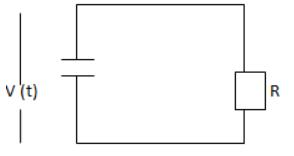Solution:

In the circuit shown voltage across capacitor decays exponentially with time at a rate determined by time constant RC. Hence the larger the resistor, the slower will be the rate of decay of v (t) with time.

QUESTION: 2

### Which of the passive component performs differentiation operation?

Solution:
• Inductor performs differentiation.
• It is given by y (t) = L d i(t) / dt where, I (t) denotes a current flowing through an inductor of inductance L.
QUESTION: 3

###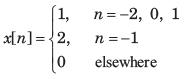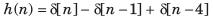Solution: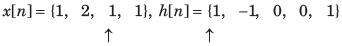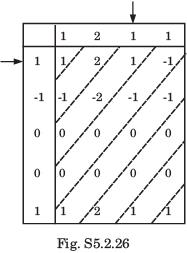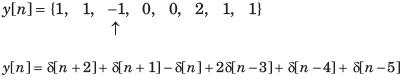QUESTION: 4

The time period of the continuous-time sinusoidal signal is given by _____.

Solution:

X (t) = A cos (wt + φ) is the continuous-time sinusoidal signal and its period is given by
T = 2π / w where w is the frequency in radians per second.

QUESTION: 5

In question y[n] is the convolution of two signal. Choose correct option for y[n]. y[n] =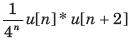Solution:

For n + 2<0 or n < -2, y [n] = 0
For n + 2 ≥ 0 or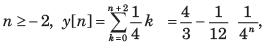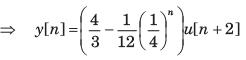QUESTION: 6

In question y[n] is the convolution of two signal. Choose correct option for y[n].
y[n] = 3n u [-n + 3] * u[n - 2]

Solution: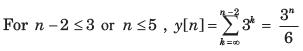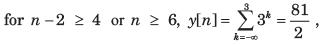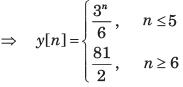QUESTION: 7

In question y[n] is the convolution of two signal. Choose correct option for y[n].
y[n] = u [n + 3] * u[n-3]

Solution:

For n - 3 < -3 or n < 0, y[n] = 0
for n - 3 ≥ -3  or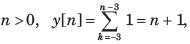y[n] = ( n + 1) u[ n]

QUESTION: 8

The convolution of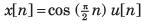and h[n] = u[n - 1] is f [ n]u[n - 1]. The function f [n] is:

Solution:

For n - 1<0 or n < 1 , y[n] = 0
For n - 1 ≥ 0 or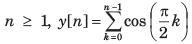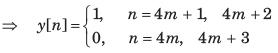QUESTION: 9

Let P be linearity, Q be time invariance, R be causality and S be stability. In question discrete time input x[n] and output y[n] relationship has been given. In the option properties of system has been given. Choose the option which match the properties for system.
Q. y[ n] = rect ( x[ n])

Solution: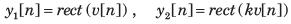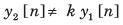(Not Homogeneous not linear)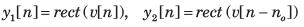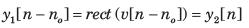(Time Invariant) At any discrete time n  = no , the response depends only on the excitation at that discrete time.
(Causal) No matter what values the excitation may have the response can only have the values zero or one.

QUESTION: 10

Let P be linearity, Q be time invariance, R be causality and S be stability. In question discrete time input x[n] and output y[n] relationship has been given. In the option properties of system has been given. Choose the option which match the properties for system.
Q. y[n] = nx[n]

Solution: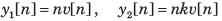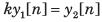(Homogeneous)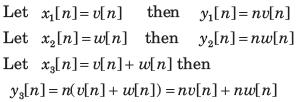= y1[n] + y2 [n] (Additive)
Since the system is homogeneous and additive, it is also linear.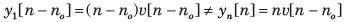(Time variant)
At any discrete time, n = no the response depends only on the excitation at that same time. (Causal)
If the excitation is a constant, the response is unbounded as n approaches infinity. (Unstable)